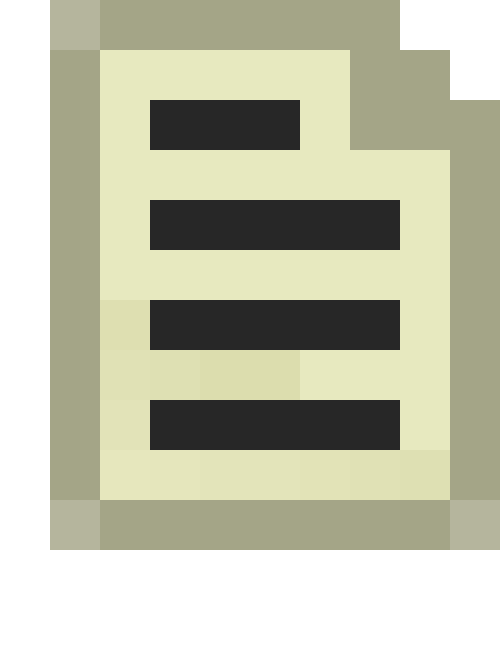# AngleVectors() and VectorAngles()

`AngleVectors()` converts a `QAngle` into either one or three normalised `Vectors`.

• If there is only one Vector, it points in the same direction as the QAngle ("forward").
• If there are three Vectors, they point forward, right and up respectively.

`VectorAngles()` converts a single `Vector` into a `QAngle`. You can optionally pass a `pseudoup Vector` which is useful if you're creating the QAngle based on orthogonal axes and don't want to lose rotational information; it will merely determine the roll component of the QAngle.Note:None of the functions return values; instead, you must declare all the variables you need beforehand. `AngleVectors()` requires you to pass pointers while `VectorAngles()` does not.

## Example

```Vector fwd, rt, up;
AngleVectors( myAngle, &fwd, &rt, &up);
```
```QAngle angles;
VectorAngles( myVector, angles );
```